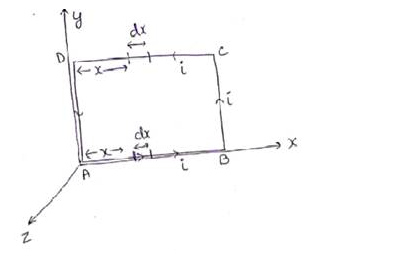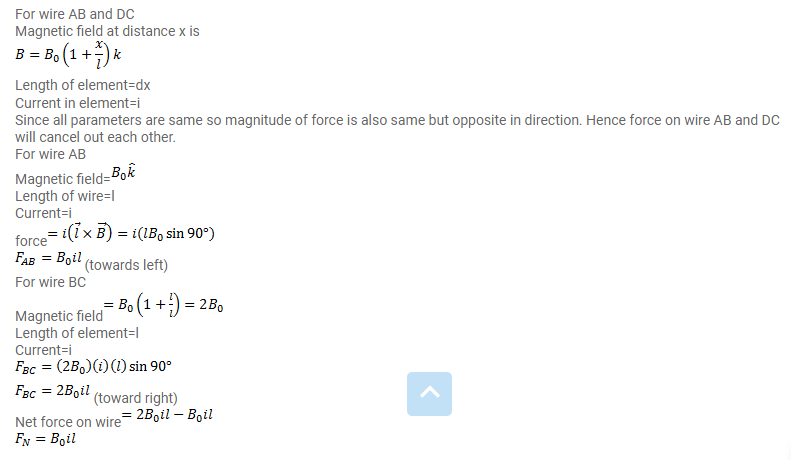# The magnetic field existing in a region is given by

Question:

The magnetic field existing in a region is given by $\vec{B}=B_{0}\left(1+\frac{x}{l}\right) \vec{k}$

A square loop of edge $I$ and carrying a current $i$, is placed with its edge parallel to the $X-Y$ axes. Find the magnitude of the net magnetic force experienced by the loop.

Solution: